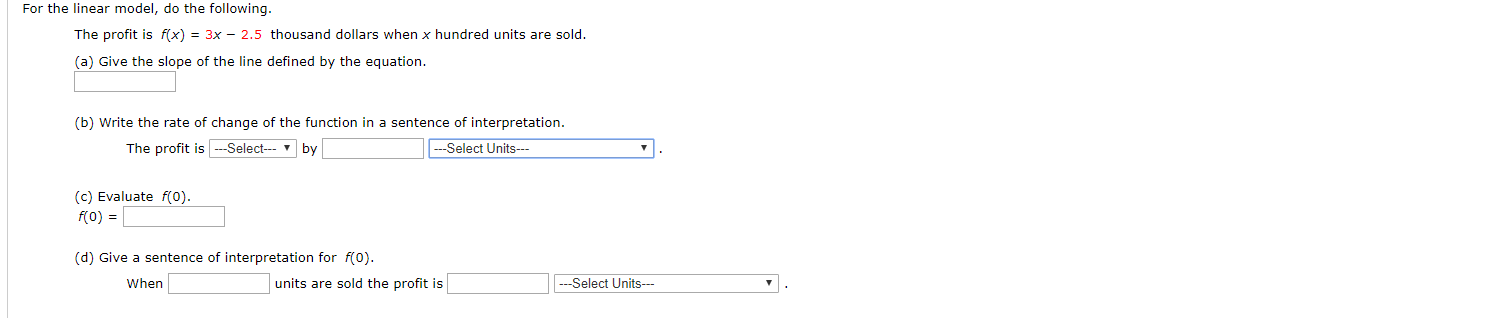# For the linear model, do the following.The profit is f(x) = 3x - 2.5 thousand dollars when x hundred units are sold.(a) Give the slope of the line defined by the equation(b) Write the rate of change of the function in a sentence of interpretation.---Select Units-The profit is-Selectby(c) Evaluate f(0).f(0)(d) Give a sentence of interpretation for f(0).--Select Units-Whenunits are sold the profit is

Question
4 viewshelp_outlineImage TranscriptioncloseFor the linear model, do the following. The profit is f(x) = 3x - 2.5 thousand dollars when x hundred units are sold. (a) Give the slope of the line defined by the equation (b) Write the rate of change of the function in a sentence of interpretation. ---Select Units- The profit is-Select by (c) Evaluate f(0). f(0) (d) Give a sentence of interpretation for f(0). --Select Units- When units are sold the profit is fullscreen
check_circle

Step 1

Since we only answer up to 3 sub-parts, we’ll answer the first 3. Please resubmit the question and specify the other subparts (up to 3) you’d like answered.

We compare the line with f(x)=mx+b.

So, m=3

Step 2

(b) Since 3 is positive the function is increasing. And f(x) is in thousands. So f(x)=3 means 3000 dollars. And x is in hu...

### Want to see the full answer?

See Solution

#### Want to see this answer and more?

Solutions are written by subject experts who are available 24/7. Questions are typically answered within 1 hour.*

See Solution
*Response times may vary by subject and question.
Tagged in

### Other